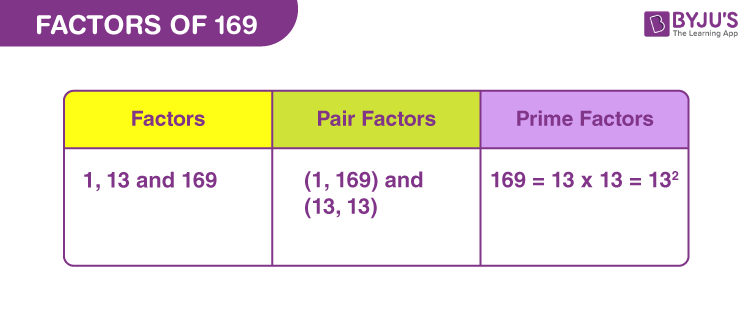# Factors of 169

Factors of 169 are the natural numbers that can divide the original number into equal parts. If the product of two natural numbers is 169, then the two numbers are the required factors. 169 is the perfect square of 13, since, 13 x 13 = 169 and 13 is an odd prime number. Also, 169 is a composite numberThe pair factors of 169 can be expressed in positive form or negative form. For example, the pair factors of 169 are represented as (1, 169) or (-1, -169). If we multiply a pair of negative numbers, such as multiplying -1 and -169, we will get the original number.In this article, we are going to discuss the what are the factors of 169, positive and negative pair factors of 169, and the prime factorisation of 169 with many solved examples.

## What are the Factors of 169?

The factors of 169 are the numbers that divide the number 169 without leaving any remainder. As the number 169 is a composite number, it has more than two factors. Thus, the factors of 169 are 1, 13 and 169. Similarly, the negative factors of 169 are -1, -13 and -169.

 Factors of 169: 1, 13 and 169. Prime Factorisation of 169: 13 × 13 or 132.

## Pair Factors of 169

The pair factors of 169 will result in the original number when multiplied together. Since, we have already figured out that there are only three factors, 1, 13 and 169, thus, we can find the factors in pair as:

1 × 169 = 169

13 × 13 = 169

Therefore, the positive pair factors are (1, 169) and (13, 13).

Now, if we consider negative pair factors then the multiplication of two negative numbers will result in the original number.

-1 × -169 = 169

-13 × -13 = 169

Therefore, the negative pair factors are (-1, -169) and (-13, -13).

## How to Find the Factors of 169?

Factors of 169 are the integers that divide the original number, evenly. When 169 is divided by its factor, then the remainder is zero. Thus, we can find the factors by the division method.

169 ÷ 1 = 169

169 ÷ 13 = 13

169 ÷ 169 = 1

Thus, we can conclude that only 1, 13 and 169 are the factors of 169.

## Prime Factorisation of 169

The prime factorisation of 169 is the method of writing the number 169 as the product of its prime factors. To find the prime factors of 169, go through the procedure given below.

Consider a pair factor of 169, say (1, 169).

Here, the number 1 is neither prime nor composite. Hence, ignore the number 1. Now, consider the other number 169, which is a composite number and can be split further into its prime factors.

Thus, the number 169 is written as the product of 13 and 13.

Therefore, the prime factorisation of 169 = 13 x 13 or 132.

### Solved Examples

Q.1: Neeta brought 13 pens for Rs.169. What is the cost of each pen?

Solution: Given,

Number of pens = 13

Cost of 13 pens = Rs.169

Cost of each pen = 169/13 = Rs.13

Q.2: What is the sum of all the factors of 169?

Solution: The factors of 169 are 1, 13, and 169.

Sum = 1+13+169 = 183

Therefore, 183 is the required sum.

Q.3: What are the common factors of 117 and 169?

Solution: Let us list the factors of both the numbers 117 and 169.

117 → 1, 3, 9, 13, 39, 117

169 → 1, 13, 169.

Therefore, the common factors of 117 and 169 are 1 and 13.

Q.4: Find the GCF of 169 and 180?

Solution: The individual factors are:

169 → 1, 13, 169

180 → 1, 2, 3, 4, 5, 6, 9, 10, 12, 15, 18, 20, 30, 36, 45, 60, 90, 180

Therefore,

GCF (169, 180) = 1

### Practice Questions

1. Find the common factors of 169 and 168?
2. Find the common factors of 169 and 191.
3. Is 31 a factor of 169?
4. What is the greatest common factor for 169 and 338?

## Frequently Asked Questions on Factors of 169

### How many factors of 169 are there?

There are three factors of 169. They are 1, 13, and 169.

### Is 169 also a multiple of itself?

169 multiplied by 1 is equal to 169. Therefore, 169 is also a multiple of itself.

### What is the highest factor of 169 apart from itself?

The highest factor of 169 apart from itself is 13.

### What are the multiples of 169?

The first 10 multiples of 169 are 169, 338, 507, 676, 845, 1014, 1183, 1352, 1521 and 1690.

### Is 169 a prime number?

169 is a composite number, not a prime number because it has more than two factors.

### What is the prime factor form of 169?

The prime factor form of 169 is 13 x 13.

### Is 169 a perfect square or a perfect cube?

169 is a perfect square because the square root of 169 is equal to 13.